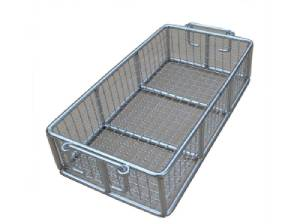Focusing on filtration screens

# Why does Stainless Steel Filter Screen Not Rust? (Part 2)

Apr. 06, 2020
Share:

Ferritic stainless steel: Stainless steel with a ferrite structure as the main component in use. The chromium content is 11% ~ 30%, and it has a body-centred cubic crystal structure. This type of steel generally does not contain nickel, and sometimes contains a small amount of elements such as Mo, Ti, Nb, etc. This type of steel has the characteristics of large thermal conductivity, small expansion coefficient, good oxidation resistance, and excellent stress corrosion resistance. , Water vapour, water and oxidizing acid parts. This kind of steel has the disadvantages of poor plasticity, significantly reduced plasticity after welding and corrosion resistance, which limits its application. The application of external furnace refining technology (AOD or VOD) can greatly reduce the gap elements such as carbon and nitrogen, so this type of steel is widely used.The physical properties of the stainless steel wire basket are mainly expressed by the following aspects:

① Coefficient of thermal expansion: The change in the element of physical quality due to the change in temperature. The expansion coefficient is the slope of the expansion-temperature curve, the instantaneous expansion coefficient is the slope at a specific temperature, and the average slope between two specified temperatures is the average thermal expansion coefficient. The expansion coefficient can be expressed by volume or length, usually by length.

② Density: The density of a substance is the mass per unit volume of the substance, and the unit is kg / m3 or 1b / in3.

③. Elastic Modulus: When a force is applied to both ends of a unit length edge to cause a unit change in length of an object, the force required per unit area is called the elastic modulus. The unit is 1b / in3 or N / m3.

④ Resistivity: The resistance measured between two opposite sides of a unit length cube material.

⑤. Permeability: Dimensionless coefficient, which indicates the degree to which a substance is easily magnetized. It is the ratio of magnetic induction strength to magnetic field strength.

⑥. Melting temperature range: Determine the temperature at which the alloy begins to solidify and complete.

⑦. Specific heat: The amount of heat required to change the temperature of a substance per unit mass by 1 degree. The values of specific heat are the same in the British and CGs systems because the unit of heat (Biu or cal) depends on the unit of water required to raise the heat by 1 degree. The specific heat value in the international unit system is different from the English or CGS system because the unit of energy (J) is defined according to different definitions.

⑧. Thermal conductivity: A measure of the rate at which a substance conducts heat. When a temperature gradient of 1 degree per unit length is established on a unit cross-sectional area, then the thermal conductivity is defined as the amount of heat conducted per unit time.

⑨. Thermal diffusivity: It is a property that determines the temperature advance rate of the internal temperature of a substance. It is the ratio of the thermal conductivity to the product of heat and density.